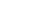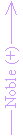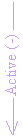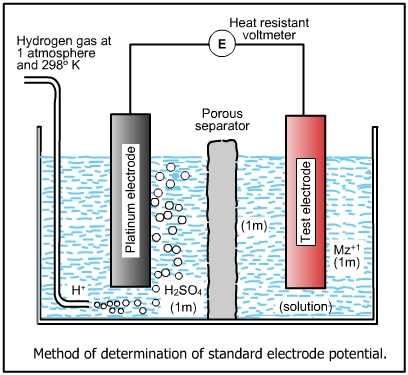2. Understanding Corrosion 2.3 Electromotive Series [1/2]

In an electromotive force series (Table 2.1), the potentials of pure metals are listed in accordance with their tendencies to corrode, the metals with the most positive potentials on the top  (like Ag, Au, Pt, etc.) followed by metals with negative potentials in the decreasing order (Na, Mg, K, etc.). The potentials listed in the table given below are called "Standard Reduction Potentials" as the half cell reaction are written in the reduction form (Au3+ + 3e-Au). Chemists generally use the emf series in which potentials are written as oxidation reactions (AuAu3+ + 3e-), and they are termed as "Standard Oxidation Potentials". The standard potentials (Eo) are measured against a standard hydrogen electrode which is at 1 atm pressure and the H+ concentration is 1 molar. The standard hydrogen electrode has been assigned a potential of 0 V at 25oC.

Table 2.1: Standard Oxidation-Reduction Potentials, Volts (25oC, against Normal Hydrogen Electrode)AuAu3+ + 3e + 1.498 O2 + 4H+ + 4e2H2O + 1.229 PtPt2+ + 2e + 1.200 PdPd2+ + 2e + 0.987 AgAg+ + e + 0.799 2HgHg2+ + 2e + 0.788 FeFe3+ + 3e + 0.771 O2 + 2H2O + 4e4OH- + 0.401 CuCu2+ + 2e + 0.337 Sn4+ + 2eSn2+ + 0.150 2H+ + 2eH2 0.000PbPb2+ + 2e - 0.126 SnSn2+ + 2e - 0.135 NiNi2+ + 2e - 0.250 CoCo2+ + 2e - 0.277 CdCd2+ + 2e - 0.403 FeFe2+ + 2e - 0.440 CrCr2+ + 2e - 0.744 ZnZn2+ + 2e - 0.763 AlAl3+ + 3e - 1.662 MgMg2+ + 2e - 2.363 NaNa+ + e - 2.714 KK+ + e - 2.925

The method of measurement is shown below.